Courses

# Olympiad Test : Figures Puzzles -2

## 10 Questions MCQ Test National Cyber Olympiad Class 7 | Olympiad Test : Figures Puzzles -2

Description
This mock test of Olympiad Test : Figures Puzzles -2 for Class 7 helps you for every Class 7 entrance exam. This contains 10 Multiple Choice Questions for Class 7 Olympiad Test : Figures Puzzles -2 (mcq) to study with solutions a complete question bank. The solved questions answers in this Olympiad Test : Figures Puzzles -2 quiz give you a good mix of easy questions and tough questions. Class 7 students definitely take this Olympiad Test : Figures Puzzles -2 exercise for a better result in the exam. You can find other Olympiad Test : Figures Puzzles -2 extra questions, long questions & short questions for Class 7 on EduRev as well by searching above.
QUESTION: 1

### In this type of question, a figure or a matrix is given in which some numbers are filled according to a rule. A place is left blank.You have to find out a character (a number or a letter) from the given possible answers which may be filled in the blank space. Q. Which one will replace the question mark?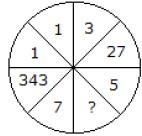Solution:

All numbers are cubed,
(7)3 = 343
(1)3 = 1
(3)3 = 27
Similarly, (5)3 = 125

QUESTION: 2

### In this type of question, a figure or a matrix is given in which some numbers are filled according to a rule. A place is left blank.You have to find out a character (a number or a letter) from the given possible answers which may be filled in the blank space. Q. Which one will replace the question mark?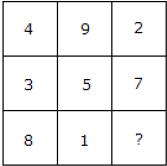Solution:

(4 + 9 + 2) = (3 + 5 + 7) = (8 + 1 + 6)
Total in each case = 15

QUESTION: 3

### In this type of question, a figure or a matrix is given in which some numbers are filled according to a rule. A place is left blank.You have to find out a character (a number or a letter) from the given possible answers which may be filled in the blank space. Q. Which one will replace the question mark?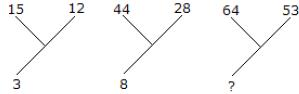Solution:

(15 + 12)/9 = 3
and (44 + 28)/9 = 8
Therefore, (64 + 53)/9 = 13

QUESTION: 4

In this type of question, a figure or a matrix is given in which some numbers are filled according to a rule. A place is left blank.You have to find out a character (a number or a letter) from the given possible answers which may be filled in the blank space.
Q. Which one will replace the question mark?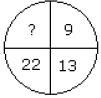Solution:

9 + (2)2 = 13
13 + (3)2 = 22
22 + (4)2 = 38

QUESTION: 5

In this type of questions, a figure or a matrix is given in which some numbers are filled according to a rule. A place is left blank.You have to find out a character (a number or aletter) from the given possible answers which maybe filled in the blank space.
Q. Which one will replace the question mark?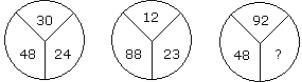Solution:

(30 – 24) × 8 = 48
and (23 – 12) × 8 = 88
Therefore, (92 – 86) × 8 = 48

QUESTION: 6

In this type of question, a figure or a matrix is given in which some numbers are filled according to a rule. A place is left blank.You have to find out a character (a number or a letter) from the given possible answers which may be filled in the blank space.
Q. Which one will replace the question mark?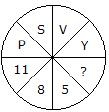Solution:

Putting the position of the letters in reverse
order
P = 11, S = 8, V = 5 and Y = 2

QUESTION: 7

In this type of question, a figure or a matrix is given in which some numbers are filled according to a rule. A place is left blank.You have to find out a character (a number or a letter) from the given possible answers which may be filled in the blank space.
Q. Which one will replace the question mark?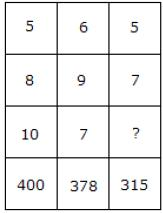Solution:

5 × 8 × 10 = 400
and 6 × 9 × 7 = 378
Therefore 5 × 7 × ? = 315
? = 9

QUESTION: 8

In this type of question, a figure or a matrix is given in which some numbers are filled according to a rule. A place is left blank.You have to find out a character (a number or a letter) from the given possible answers which may be filled in the blank space.
Q. Which one will replace the question mark?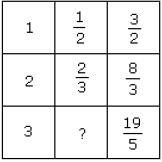Solution:

From I row, 1 + (1/2) = 3/2
From II row, 2 + (2/3) = 8/3
From III row, 3 + ? = 19/5
? = (19/5) – 3
? = (4/5)

QUESTION: 9

In this type of questions, a figure or a matrix is given in which some numbers are filled according to a rule. A place is left blank.You have to find out a character (a number or aletter) from the given possible answers which maybe filled in the blank space.
Q. Which one will replace the question mark?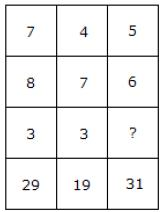Solution:

(7 × 3) + 8 = 29
(4 × 3) + 7 = 19
(5 × ?) + 6 = 31
? = 5.

QUESTION: 10

In this type of questions, a figure or a matrix is given in which some numbers are filled according to a rule. A place is left blank.You have to find out a character (a number or aletter) from the given possible answers which maybe filled in the blank space.
Q. Which one will replace the question mark?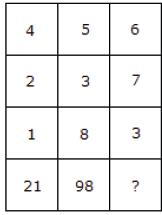Solution:

(4)2 + (2)2 + (1)2 = 21
and (5)2 + (3)2 + (8)2 = 98
Therefore, (6)2 + (7)2 + (3)2 = 94